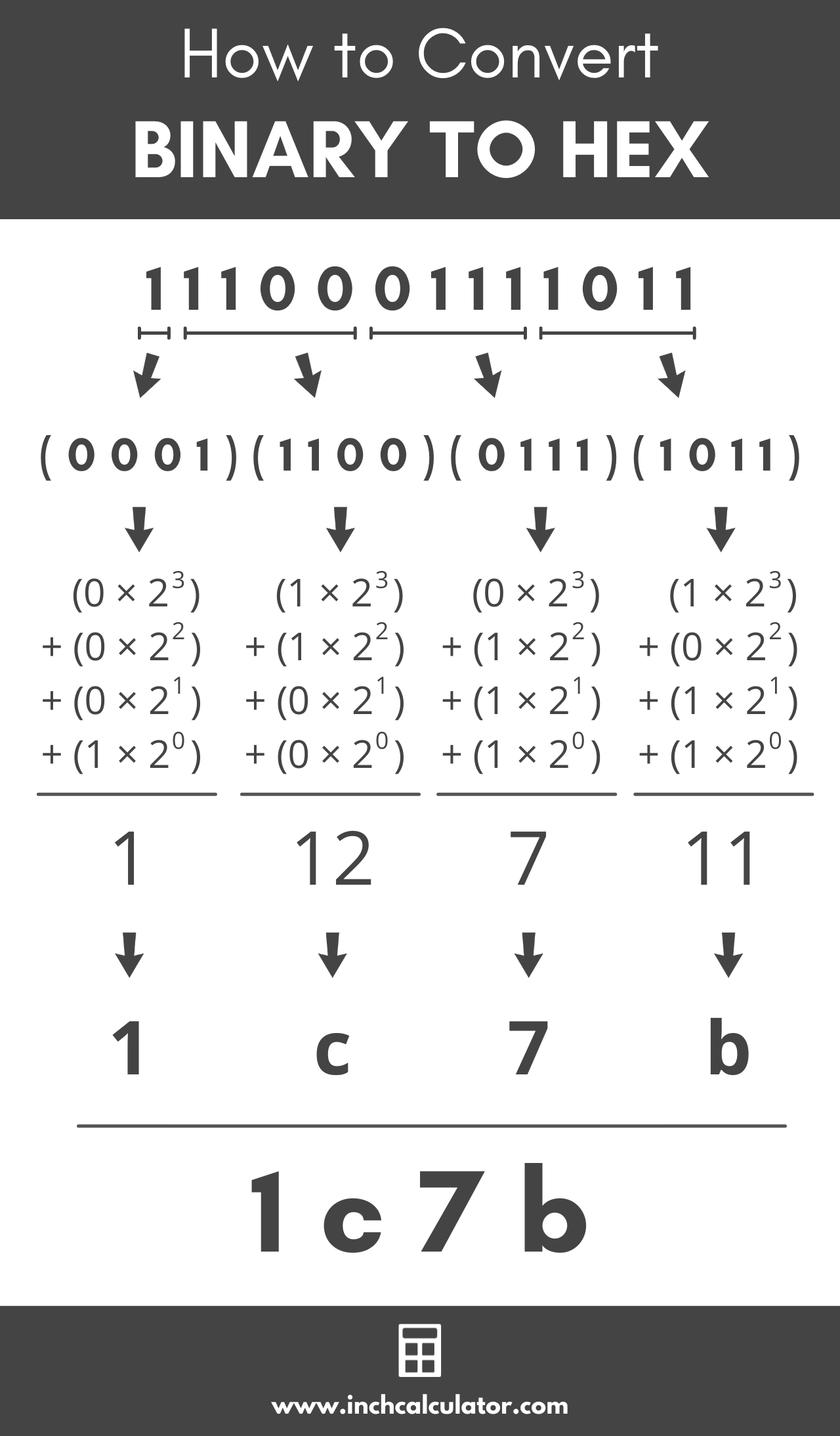Enter a binary number below to convert it to hex.

### Steps to Convert to Hexadecimal

Learn how we calculated this below

## How to Convert Binary to Hex

The binary number system is a base 2 number system since it only uses the digits 0 and 1. Hexadecimal, also known as hex, is a base 16 number system since it uses sixteen digits, 0 to 9 as well as the letters A through F.

Binary and hexadecimal numbers are often used in computing, networking, and software applications, so it is fairly common to need to convert from one to the other.

You can convert from binary to hex in a few simple steps.

### Step One: Split into Groups of Four Digits

The first step is to break the binary number into groups of four digits, starting from the right and moving to the left. The reason for this is that a group of four base 2 numbers, or 24 is equal to 16, which is evenly divisible into the base 16 number system.

For example, the binary number 1110001111011 can be broken into the following groups:

1110001111011
(1)(1100)(0111)(1011)

It is okay if the first group does not have four digits. You can also add additional zeros to precede the digits in the first group so that there are four digits.

### Step Two: Convert Each Binary Group to a Hexadecimal Digit

At this point, each group of four binary digits can be converted to a hexadecimal digit.

12 = 110 = 116
11002 = 8 + 4 + 0 + 0 = 1210 = c16
01112 = 0 + 4 + 2 + 1 = 710 = 716
10112 = 8 + 0 + 2 + 1 = 1110 = b16

So, 11100011110112 in binary is equal to 1c7b16 in hex.

### Binary Nibble Hex Values

The following table shows the hex digits for each possible group, also called a nibble, of four binary digits.

Binary nibbles converted to the equivalent hex digits
0000 0
0001 1
0010 2
0011 3
0100 4
0101 5
0110 6
0111 7
1000 8
1001 9
1010 a
1011 b
1100 c
1101 d
1110 e
1111 fYou can also use a tool like our binary calculator to convert to decimal or hex.

### Binary to Hex Conversion Table

The table below shows binary numbers and the equivalent hexadecimal number values.

Binary numbers converted to the equivalent hex values
Binary Number Hexadecimal Number Decimal Number
0 0 0
1 1 1
10 2 2
11 3 3
100 4 4
101 5 5
110 6 6
111 7 7
1000 8 8
1001 9 9
1010 a 10
1011 b 11
1100 c 12
1101 d 13
1110 e 14
1111 f 15
10000 10 16
10001 11 17
10010 12 18
10011 13 19
10100 14 20
10101 15 21
10110 16 22
10111 17 23
11000 18 24
11001 19 25
11010 1a 26
11011 1b 27
11100 1c 28
11101 1d 29
11110 1e 30
11111 1f 31
100000 20 32
1000000 40 64
10000000 80 128
100000000 100 256
1000000000 200 512
10000000000 400 1024
100000000000 800 2048

You might also be interested in our hex calculator to add or subtract hexadecimal numbers.